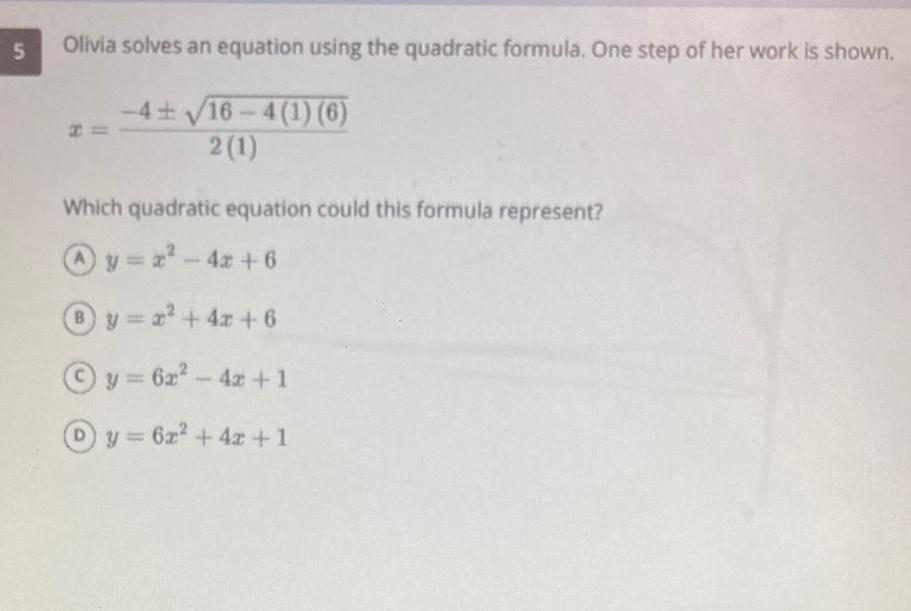Question:

# 5 Olivia solves an equation using the quadratic formula One

Last updated: 3/9/20235 Olivia solves an equation using the quadratic formula One step of her work is shown 4 16 4 1 6 2 1 Which quadratic equation could this formula represent y x 4x 6 y x 4x 6 y 6x 4x 1 Dy 6x 4x 1